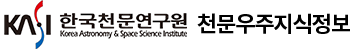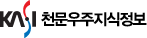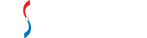#통합검색

#### 통합검색

인기검색어### 논문 상세보기

• 홈으로 이동
DECAY OF TURBULENCE IN FLUIDS WITH POLYTROPIC EQUATIONS OF STATE
• 저자
Lim, Jeonghoon
• 저널명
The Korean Astronomical Society
• 발행년도
2020
• 권호
V .53, N .2
• 원문보기
We present numerical simulations of decaying hydrodynamic turbulence initially driven by solenoidal (divergence-free) and compressive (curl-free) drivings. Most previous numerical studies for decaying turbulence assume an isothermal equation of state (EOS). Here we use a polytropic EOS, P ∝ ργ, with polytropic exponent γ ranging from 0.7 to 5/3. We mainly aim at determining the effects of γ and driving schemes on the decay law of turbulence energy, E ∝ t-α. We additionally study probability density function (PDF) of gas density and skewness of the distribution in polytropic turbulence driven by compressive driving. Our findings are as follows. First of all, we find that even if γ does not strongly change the decay law, the driving schemes weakly change the relation; in our all simulations, turbulence decays with α ≈ 1, but compressive driving yields smaller α than solenoidal driving at the same sonic Mach number. Second, we calculate compressive and solenoidal velocity components separately and compare their decay rates in turbulence initially driven by compressive driving. We find that the former decays much faster so that it ends up having a smaller fraction than the latter. Third, the density PDF of compressively driven turbulence with γ > 1 deviates from log-normal distribution: it has a power-law tail at low density as in the case of solenoidally driven turbulence. However, as it decays, the density PDF becomes approximately log-normal. We discuss why decay rates of compressive and solenoidal velocity components are different in compressively driven turbulence and astrophysical implication of our findings.